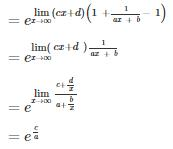Courses

# Engineering Mathematics 4

## 10 Questions MCQ Test Mock Test Series - Computer Science Engg. (CSE) GATE 2020 | Engineering Mathematics 4

Description
This mock test of Engineering Mathematics 4 for Computer Science Engineering (CSE) helps you for every Computer Science Engineering (CSE) entrance exam. This contains 10 Multiple Choice Questions for Computer Science Engineering (CSE) Engineering Mathematics 4 (mcq) to study with solutions a complete question bank. The solved questions answers in this Engineering Mathematics 4 quiz give you a good mix of easy questions and tough questions. Computer Science Engineering (CSE) students definitely take this Engineering Mathematics 4 exercise for a better result in the exam. You can find other Engineering Mathematics 4 extra questions, long questions & short questions for Computer Science Engineering (CSE) on EduRev as well by searching above.
QUESTION: 1

### Find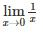Solution: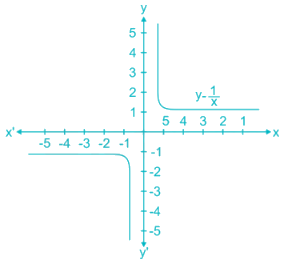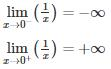Since the limit from the left does not equal to the limit from the right. So limit does not exist.

QUESTION: 2

### If f(x) is continuous at x = 0 where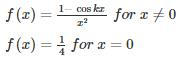then what is the value of k?

Solution:

Since given function is continuous at x = 0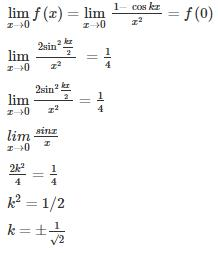Alternate method:

0/0 form using L Hospital’s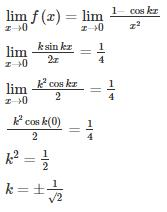QUESTION: 3

### If f(x) is continuous at x = 0 where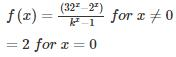then what is the value of k?

Solution:

f(x) is continuous at x = 0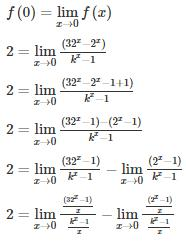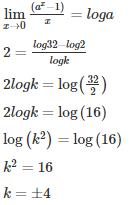By definition of logarithm

k ≠ -4

∴ k = 4

Alternate method using L Hospital's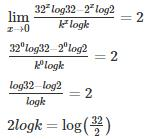2logk = log (16)

2logk = 2log (4)

∴ k = 4

*Answer can only contain numeric values
QUESTION: 4

The value of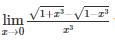will be ______

Solution: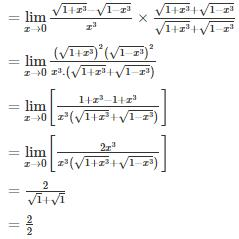= 1

QUESTION: 5

The value of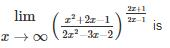Solution: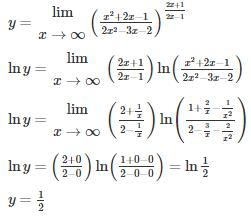QUESTION: 6

The value of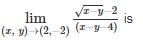Solution: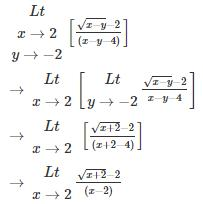Applying L-Hospital Rule

Since the limit is of the form 0/0

Therefore,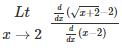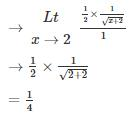*Answer can only contain numeric values
QUESTION: 7

If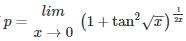Find ln p

Solution:

Concept: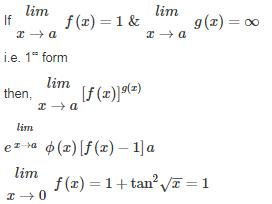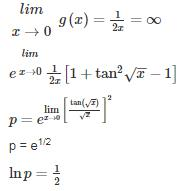QUESTION: 8

Find the value for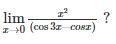Solution: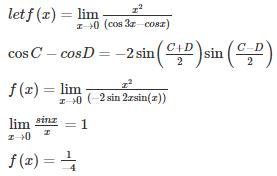Alternate method: Using L Hospital’s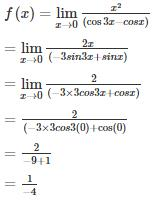QUESTION: 9

What is the value of f(x) =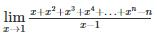Solution: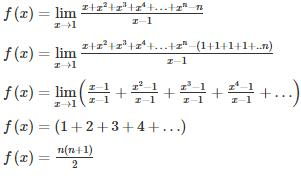QUESTION: 10

Find the value of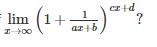Solution: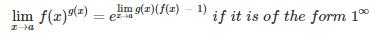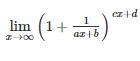Substituting x = ∞

It is of the form 1